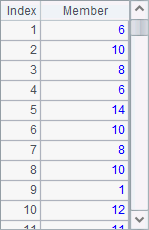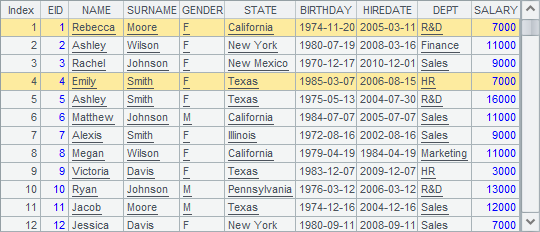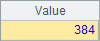# 主键与索引功能

## find 和 pfind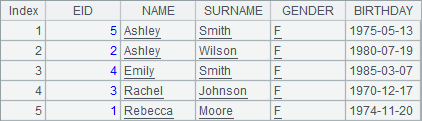A 1 =demo.query("select * from EMPLOYEE") 2 =A1.derive(NAME+" "+SURNAME:FullName) 3 =10000.(A2(rand(A2.len())+1).FullName) 4 =now() 5 =A3.(A2.pselect(FullName:A3.~)) 6 =interval@ms(A4,now()) 7 =now() 8 >A2.keys(FullName) 9 =A3.(A2.pfind(A3.~)) 10 =interval@ms(A7,now())

A5A9中基于相同的数据，分别使用pselectpfind函数在序表中查找记录的位置，其中，使用pfind之前，需要用keys函数设定序表的主键。A6A10中分别计算出耗费的毫秒数如下：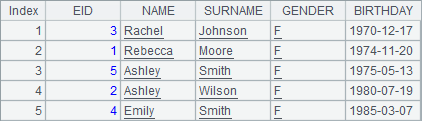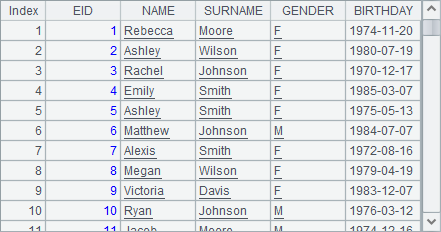A5A9中的查找结果是相同的：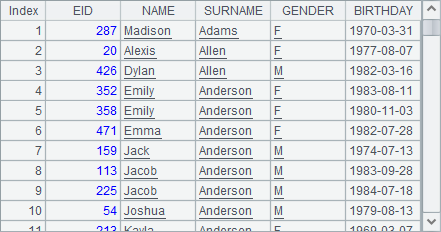A 1 =demo.query("select * from EMPLOYEE") 2 =A1.derive(NAME+" "+SURNAME:FullName) 3 =10000.(A2(rand(A2.len())+1).FullName) 4 =now() 5 =A3.(A2.select@1(FullName==A3.~)) 6 =interval@ms(A4,now()) 7 =now() 8 >A2.keys(FullName) 9 =A3.(A2.find(A3.~)) 10 =interval@ms(A7,now())

A6A10中分别计算出耗费的毫秒数如下：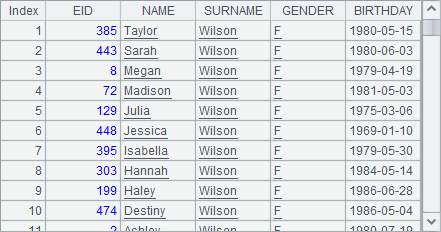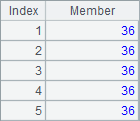A5A9中的查找结果仍然是相同的：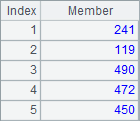## 主键与索引表

 A 1 =demo.query("select * from EMPLOYEE").keys(EID) 2 =10000.(A1(rand(A1.len())+1).EID) 3 =now() 4 =A2.(A1(A2.~)) 5 =interval@ms(A3,now()) 6 =now() 7 =A2.(A1.find(A2.~)) 8 =interval@ms(A6,now())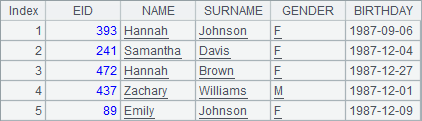## switch 函数

 A 1 =file("D:/files/txt/PersonnelInfo.btx ") 2 =A1.import@b() 3 =A1.import@b() 4 =demo.query("select * from STATES") 5 =now() 6 >A2.(State=A4.select@1(ABBR:A2.State)) 7 =interval@ms(A5,now()) 8 =now() 9 >A3.switch(State,A4:ABBR) 10 =interval@ms(A8,now())

A2A3中都是从集文件PersonnelInfo.btx中读取的人员资料，如下：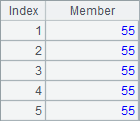A4中是州信息表，如下：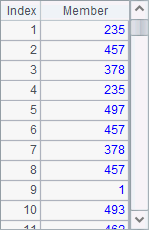A6A9中，将人员资料表中的State字段变换为对应的州信息，不同的是A6中用select@1函数，A9中则使用switch函数。在A7A10中分别计算出两种方法的耗时如下：